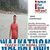## TyroCity is a community of amazing learners

We're a place where students share, stay up-to-date, learn and grow.

# Discussion on: About Marginal cost of capitalCost of Capital:

First, here I am going to discuss on cost of capital. A firm’s cost of capital is the rate the firm has to pay for the use of debt, preferred stock, common stock, and/or retained earnings. In other words it is the rate that must be earned on the firm’s investment in order to satisfy all the investors required rate of return. Cost of capital is an important concept in financial decision making. It is used as standard for evaluating investment projects, designing capital structure and determining appropriate discounting rate to compute net present value (NPV) of cash flow and to compare with internal rate of return (IRR) for evaluating worthiness of investment proposals (Michael, 2016).

A firm’s should compute component cost of capital to determine weighted average cost of capital. Before - tax cost of debt, kd, is the rate of return required by the debt holders. After - tax cost of debt, kdt, is computed by multiplying before - tax cost of debt (1 - tax rate). Cost of preferred stock, kps, is the rate of return required by the preferred stockholders. Similarly, a firm’s cost of equity capital is defined as the rate of return required by its common stock holders. Equity capital can be raised internally through retained earnings and externally through the scale of new common stock. Cost of internal equity, ks, can be computed using dividend discounting model, capital assert pricing model, and using bond - yield - plus risk premium approach. Cost of external equity, ke, is greater than the cost of internal equity because of issuances expanses.

Marginal Cost:

The marginal cost of capital is weighted average cost of capital of last rupees of new financing. It is also called weighted marginal cost of capital (WMCC). The value minimizing firm user the WMCC which is based on target capital structure to evaluate the investment proposal. The break-even point helps to calculate WMCC (Arnold, 2008). The level of financing after which the WACC shifts upward is called break point.

Historic Cost of Capital Vs. Marginal Cost of Capital:

In economics marginal cost of any item is the cost of another unit of that item. For example, marginal cost of output is the cost of adding one additional unit of output. The same concept applies to cost of capital. The marginal cost of capital is the cost of raising an additional rupees of capital. But in practice, the capital is raised in lump sum. Hence, the marginal cost of capital in finance refers to the cost incurred in raising the incremental funds (Maness, 2009). Thus, the weighted average cost of new or incremental capital is known as the marginal cost of capital.

We computed Sunrise Company’s weighted average cost of capital to be 10.6 percent. As long as sunrise keeps its capital structure constant, as long as its component costs versus cost of debt after - tax (6%), cost of preferred stock (12%) and cost of equity (14%), then it WACC will be 10.6%. This means each rupees the company raised will consist of some long-term debt, some preferred stock, and some common equity, and the cost of the whole rupees will be 10.6 % its marginal cost of capital (MACC) will be 10.6%.

Marginal cost of capital does not remain constant. As the volume of financing increase the costs of various sources of financing increase. Because suppliers of funds required greater returns in form of interest, dividend, or growth as compensation for the increased risk introduced by large volumes of new financing, other reasons of higher cost for large amount of financing are flotation costs, underpricing of the security, discount etc, Marginal cost of capital therefore an increasing function of level new financing.

References
Arnold, G. (2008). Corporate financial management. , FT Prentice Hall, Pearson Education .

Maness, S. (2009). Introduction to Corporate Finance. McGraw-Hill, New York, USA.

Michael, T. J. (2016). Do You Know Your Cost of Capital? Harvard Business Review .